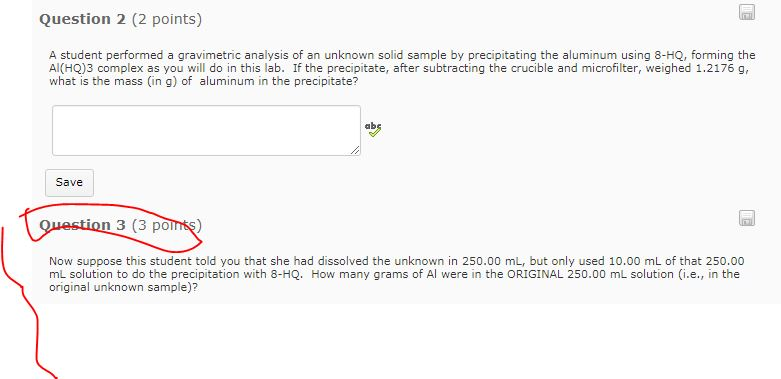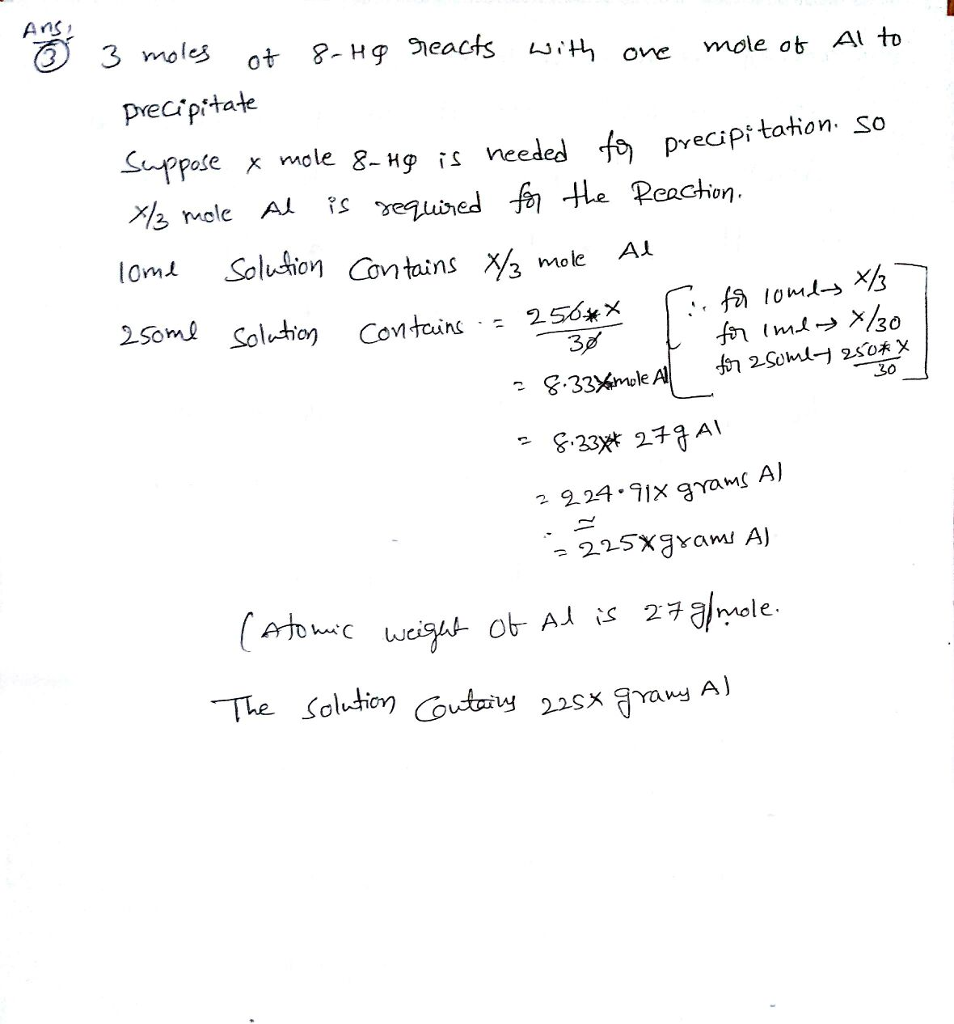# Homework Solution: Question 2 (2 points) A student performed a gravimetric analysis of an unknown solid sample…I need the answer only for question 3 but I put question 2 because maybe you need to read it to do Q3
Question 2 (2 points) A student performed a gravimetric analysis of an unknown solid sample by precipitating the aluminum using 8-HQ, forming the Al(HQ)3 complex as you will do in this lab. If the precipitate, after subtracting the crucible and microfilter, weighed 1.2176 g what is the mass (in g) of aluminum in the precipitate? ab Save ion 3 (3 po Now suppose this student told you that she had dissolved the unknown in 250.00 mL, but only used 10.00 mL of that 250.00 mL solution to do the precipitation with 8-HQ. How many grams of Al were in the ORIGINAL 250.00 mL solution (i.e., in the original unknown sample)?